# Monday August 21st

## Solar Eclipse Day

• Today there is a total Solar Eclipse; too bad I’m in California..won’t see the entire thing. However, there is a livestream, courtesy NASA! My mentor booked his ticket to Idaho about 4 months ago, because well…geek LOL.

## Finished my third assignment for my Nanodegree

• Got this response, which was really encouraging!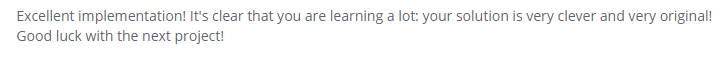• I was also recruited by yet another Valley company. It’s a student-tier, though, so I apply for a day to meet their peeps and network, etc. But I’m on their radar, and they’re a pretty large company that’s under the umbrella of a Big Four company.

## Moving on…

• Playing with Turtle!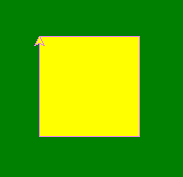• This little programme makes the line violet, and then fills the shape in yellow once it’s done!

• Yes, the turtle’s name is “brad”. calling `turtle.Turtle()` initializes the turtle.

• The window class is calling the background window and setting this.

• I’ve played around with Turtle before, and done a similar experiment in Haskell and Processing.

``````import turtle
"""
draw a square in turtle
"""

def draw_square():
window = turtle.Screen()
window.bgcolor("green")

window.exitonclick()

draw_square()
``````

## I also sent my first Twilio message!

• Awesome stuff! If I had gone a day earlier to BayHac, I would have gotten to visit their offices; a friend (from our Haskell group) works there! Now I understand a bit of what he does! Ah..so cool!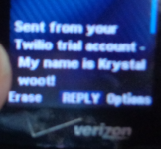• Yes, I have a flip-phone :( It says “My name is Krystal. Woot!”

## Also…went sailing!

• We made it all the way to Malibu, and saw dolphins!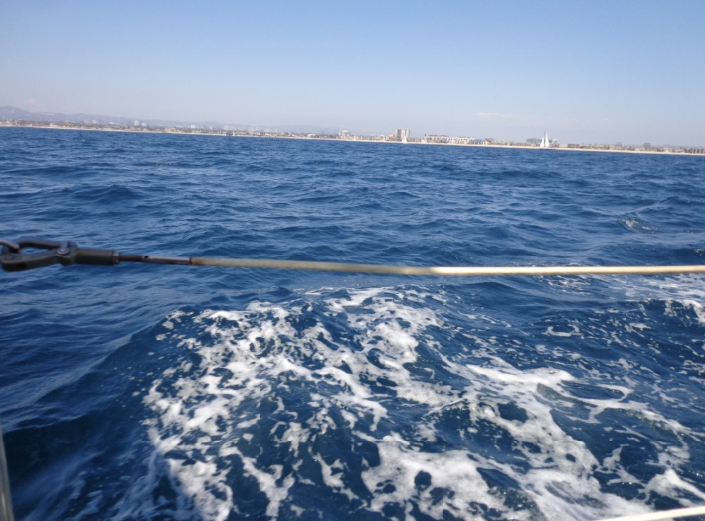## A race was going on…

• The powerboat in the middle is usually the officiating boat.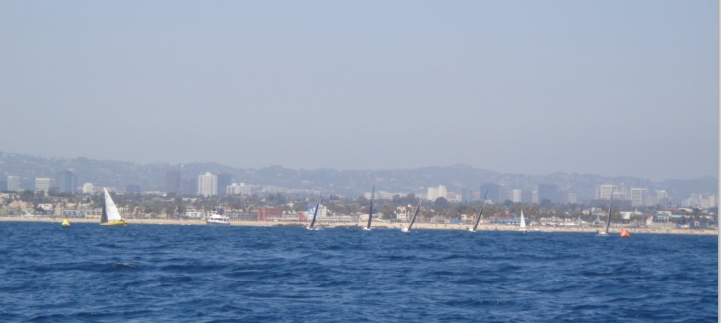## Malibu!## These guys were flying overhead

• We had an air force vet on board, so he told us what it was. I’m not that savvy, although I know a few planes (like the A-10, etc).## I had to take a photo…

• This is the name of a classmate of mine from high school! Yeah, so I went to a predominantly East-Indian descent high school…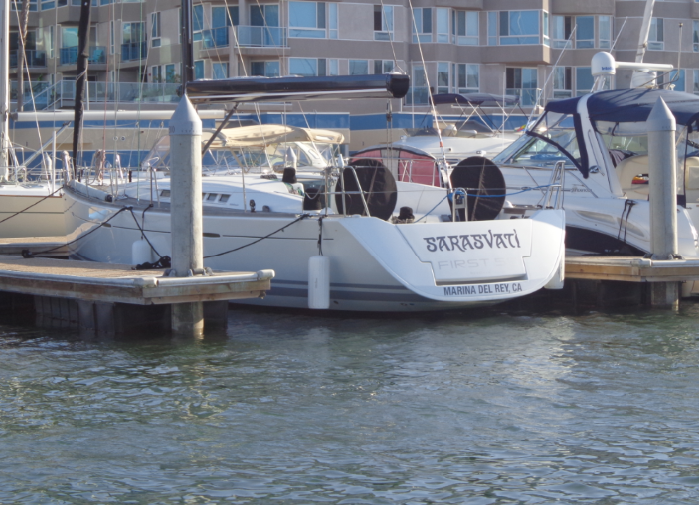## And of course…sea lions!## Plan for this week

• I’m going through the Nanodegree coursework (on to the next chapter) during the day

• In the evenings (until my class starts, on August 30th), I’ll be teaching myself some Mathematica. I have a 15-day trial, so that sounds about right, using the online version. Another tool in the toolbox that I think is very useful for what I’m interested in.

## In the top 500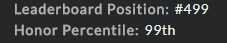## Katas solved

• Smallest value of an array
``````def find_smallest(numbers,to_return):
try:
if to_return == "value":
a = sorted(numbers)
#print a
return a
elif to_return == "index":
a = sorted(numbers)
b = a
#print numbers.index(b)
return numbers.index(b)
else:
#print None
return None
except:
#print None
return None
``````
• percentage of array. So many issues with this one. Nine reported issues, but was able to get all test cases to pass.
``````function percentageSumArray(arr){
if ((arr == null) || (arr.length) == 0){
return false
}
else{
var total = 0
var total1 = 0
var num = 0
for (var i = 0; i < arr.length; i++){
total = total + arr[i]
if (arr[i] > 0){
num = num + 1
total1 = total1 + arr[i]
}
}
var percent = ((num / 100) * total)
if (percent == 0){
return false
}
else if ((percent < 0) && (total < 0)){
return false
}
else if (isNaN(percent) == true){
return false
}
else{
var percent2 = ((num / 100 ) * total1)
var percent1 =  Math.round(percent * 100) / 100
return percent1
}
}
return
}
``````
• find vowel in string and return its index (using one-based indexing)
``````function vowelIndices(word){
var arr = []
var word1 = word.toLowerCase()
for (var i = 0; i < word1.length; i++){
if (word1[i] == 'a'){
arr.push(i+1)
}
else if (word1[i] == 'e'){
arr.push(i+1)
}
else if (word1[i] == 'i'){
arr.push(i+1)
}
else if (word1[i] == 'o'){
arr.push(i+1)
}
else if (word1[i] == 'y'){
arr.push(i+1)
}
else if (word1[i] == 'u'){
arr.push(i+1)
}
}
return(arr)
}
``````
• had to do a bunch of validations to get this to pass. I could refactor this, but I basically wanted an old kata to pass.
``````function pizzaPrice(diameter, price) {
var area = Math.PI * ((diameter / 2.0) *(diameter / 2.0))
var ppi = price * 1.0 / area * 1.0
if (ppi == 0){
return 0
}
else if ((price == undefined) || (diameter == undefined)){
return 0
}
else if (price == null){
return 0;
}
else if ((isNaN(price) === true) || (isNaN(ppi))){
return 0;
}
else if (price === NaN)
{
return 0;
}
else{
return eval(ppi.toFixed(2));
}

}
``````
• strip whitespace and compare string A and B. If A and B are equal after sorting (anagram), return true.
``````function isAnagram(stringOne, stringTwo) {
if ((stringOne == "") && (stringTwo == "")){
return true
}
else{
var a = stringOne.toLowerCase().replace(/ /g,'')
var b = stringTwo.toLowerCase().replace(/ /g,'')
var c = a.split('').sort().join('')
var d = b.split('').sort().join('')
if (c == d){
return true
}
else{
return false
}
}
return
}
``````
• Summy (has a 0 out of 4 % satisfaction rating as I write this). What they wanted was sort of confusing, but was able to pass tests.
``````def summy(String):
try:
total = 0
for i in String:
total = total + int(i)
except:
total = 0
a = String.split(' ')
for i in a:
total = total + int(i)
``````
• replace every instance or conjugated version of ‘meep’ (lowercase or uppercase) with ‘beep’
``````function meepOut(quote) {
quote = quote.replace(/meep/g, "beep")
quote = quote.replace(/Meep/g, "Beep")
return quote
}
``````
• Sort string index by evens and then odds, then join strings
``````function sortMyString(S) {

var arr = []
var arr1 = []
for (var i = 0; i < S.length; i++){
if (i % 2 == 0){
arr.push(S[i])
}
else{
arr1.push(S[i])
}
}
var a = arr.join("")
var b = arr1.join("")
var d = (a + " " + b)
return d
}
``````
• Get the integer part and separate the fraction * 8 slices
``````def how_many_pizzas(n):
a = 64.0
c = n * n
b = (c / a)

d = list(str(b))
d1 = str(b)

integer = int(b)

length = len(d)
finddot = d.index('.')

secondpart = d1[(finddot):length]

fraction = float(secondpart) * 8
fraction1 = str(fraction)
fractionfirst = fraction1

return 'pizzas: {}, slices: {}'.format(integer, fractionfirst)
``````
Written on August 21, 2017